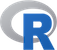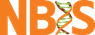@# Loops in R

In programming languages loop structures, either with or without conditions, are used to repeat commands over multiple entities. For and while loops as well as if-else statements are also often used in R, but not as often as in many other programming languages. The reason for this is that many needs of loops are solved using vectorization or via apply functions.

This means that we can multiply all values in a vector in R by two, just call:

``````vec.a <- c(1, 2, 3, 4)
vec.a * 2

 2 4 6 8
``````

In many other and languages as well as in R you can also solve this problem using a for loop

``````for (i in vec.a) {
v[i] <- vec.a[i] * 2
}
vec.a

 2 4 6 8
``````

As you saw in the lecture this is far less efficient and not by any means easier to type so we tend avoid loops when possible.

After this exercise you should know:

• What are the most common loop structures in R
• Some common alternatives to using loops in R
• How one can convert a short script to a function.
• Use that new function in R.

## Exercises: Loop structures

1. Create a 100000 by 10 matrix with the numbers 1:1000000. Make a for-loop that calculates the sum for each row of the matrix. Verify that your results are consistent with what you obtain with the apply function to calculate row sums as well as with the built-in rowSums function. These functions were discussed in the lecture “Elements of the programming language - part 2”Click to see how
```    X <- matrix(1:1000000, nrow = 100000, ncol = 10)
for.sum <- vector()
# Note that this loop is much faster if you outside the loop create an empty vector of the right size.
# rwmeans <- vector('integer', 100000)
for (i in 1:nrow(X)) {
for.sum[i] <- sum(X[i,])
}

 4500010 4500020 4500030 4500040 4500050 4500060
```Click to see Apply
```    app.sum <- apply(X, MARGIN = 1, sum)

 4500010 4500020 4500030 4500040 4500050 4500060

```Click to see RowSums
```    rowSums.sum <- rowSums(X)

 4500010 4500020 4500030 4500040 4500050 4500060

```Click to see how to check if methods are generating the same res.
```
identical(for.sum, app.sum)
identical(for.sum, rowSums.sum)
identical(for.sum, as.integer(rowSums.sum))

 TRUE
 FALSE
 TRUE

```

2. Another common loop structure that is used is the while loop, which functions much like a for loop, but will only run as long as a test condition is TRUE. Modify your for loop from exercise 1 and make it into a while loop.Click to see how
```    x <- 1
while.sum <- vector("integer", 100000)
while (x < 100000) {
while.sum[x] <- sum(X[x,])
x <- x + 1
}

 4500010 4500020 4500030 4500040 4500050 4500060

```

3. Create a data frame with two numeric and one character vector. Write a loop that loops over the columns and reports the sum of the column values if it is numeric and the total number of characters if it is a character vector.Click to see how
```
vector1 <- 1:10
vector2 <- c("Odd", "Loop", letters[1:8])
vector3 <- rnorm(10, sd = 10)
df1 <- data.frame(vector1, vector2, vector3, stringsAsFactors = FALSE)
sum.vec <- vector()
for (i in 1:ncol(df1)) {
if (is.numeric(df1[,i])) {
sum.vec[i] <- sum(df1[,i])
} else {
sum.vec[i] <- sum(nchar(df1[,i]))
}
}
sum.vec

  55.00000 15.000000  2.727954
```

4. In question 3 you generated a loop to go over a data frame. Try to convert this code to a function in R. The function should take a single data frame name as argument.Click to see how
```    df.info <- function(df) {
sum.vec <- vector()
for (i in 1:ncol(df)) {
if (is.numeric(df[,i])) {
sum.vec[i] <- mean(df[,i])
} else {
sum.vec[i] <- sum(nchar(df[,i]))
}
}
sum.vec
}
```

5. Read up on the ifelse function in R. If possible use the ifelse function to answer question 3.

6. In all loops that we tried out we have created the variable where the output is saved outside the loop. Why is this?

7. Advanced exercise At the lecture an approach to calculate factorials were implemented using recursion (function calling itself). Here we instead will have a go at generating Fibonacci numbers. A fibonacci number is part of a series of numbers with the following properties:

The first two numbers in the Fibonacci sequence are either 1 and 1, or 0 and 1, depending on the chosen starting point of the sequence, and each subsequent number is the sum of the previous two. Hence:

0, 1, 1, 2, 3, 5, 8, 13, 21, … or 1, 1, 2, 3, 5, 8, 13, 21, …

Try to generate such a series using a recursive approach